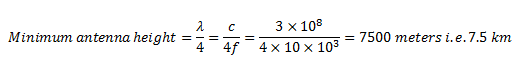# Explain the need for modulation in a communication system

## Modulation

In the modulation process, two signals are used namely the modulating signal and the carrier .

The modulating signal is nothing but the baseband signal or information signal while the carrier is a high frequency sinusoidal signal .

In the modulation process, some parameter of the carrier wave (such as amplitude, frequency or phase ) is varied in accordance with the modulating signal . This modulated signal is then transmitted by the transmitter .

The receiver demodultes the received modulated signal and gets the original information signal back .

Thus, demodulation is exactly opposite to modulation .

In the process of modulation the carrier wave actually acts as carrier which carries the information signal from the transmitter to receiver .

## Need of Modulation

You  may be ask, when the baseband signal can be transmitted directly why to use the modulation ?

The answer is that the baseband transmission has many limitations which can be overcome using modulation . It is explained below .

In the process of modulation, the baseband signal is translated i.e., shifted from low frequency to high frequency . This frequency shift is proportional to the frequency of carrier.

1. Reduction in the height of antenna
2. Avoids mixing of signals
3. Increases the range of communication
4. Multiplexing is possible
5. Improves quality of reception

We will discuss each of these advantages in detail below .

### 1. Reduction in the height of antenna

For the transmission of radio signals, the antenna height must be multiple of λ/4 ,where λ is the wavelength .

λ = c /f

where c : is the velocity of light

f: is the frequency of the signal to be transmitted

The minimum antenna height required to transmit a baseband signal of  f = 10 kHz is calculated as follows :The antenna of this height is practically impossible to install .

Now, let us consider a modulated signal at f = 1 MHz . The minimum antenna height is given by,This antenna can be easily installed practically . Thus, modulation reduces the height of the antenna .

### 2.    Avoids mixing of signals

If the baseband sound signals are transmitted without using the modulation by more than one transmitter, then all the signals will be in the same frequency range i.e. 0 to 20 kHz . Therefore, all the signals get mixed together and a receiver can not separate them from each other .

Hence, if each baseband sound signal is used to modulate a different carrier then they will occupy different slots in the frequency domain (different channels).  Thus, modulation avoids mixing of signals .

### 3.    Increase the Range of Communication

The frequency of baseband signal is low, and the low frequency signals can not travel long distance when they are transmitted . They get heavily attenuated .

The attenuation reduces with increase in frequency of the transmitted signal, and they travel longer distance .

The modulation process increases the frequency of the signal to be transmitted  . Therefore, it increases the range of communication.

### 4.    Multiplexing is possible

Multiplexing is a process in which two or more signals can be transmitted over the same communication channel simultaneously .

This is possible only with modulation.

The multiplexing allows the same channel to be used by many signals . Hence, many TV channels can use the same frequency range, without getting mixed with each other or different frequency signals can be transmitted at the same time .

### 5.  Improves Quality of Reception

With frequency modulation (FM) and  the digital communication techniques such as PCM, the effect of noise is reduced to a great extent . This improves quality of reception .

Please subscribe to Electronics Post Channel if you like my tutorials.